Sorting data in parallel CPU vs GPU

Posted on February 4, 2013 by Paul

The code for this post is on GitHub: https://github.com/sol-prog/Sort_data_parallel.

For many programmers sorting data in parallel means implementing a state of the art algorithm in their preferred programming language. However, most programming languages have a good serial sorting function in their standard library. It appears to me, that the obvious thing to do is to first try to use what your language library provides. If this approach is not successful, you should try to find an existing library that is used, and consequently well debugged, by other programmers. Only as a last resort, you should implement a new sorting algorithm from scratch.

In the case of C++, we have a well tested sorting function in the STL, std::sort, unfortunately std::sort will use only a fraction of the processing power available in a modern multicore system.

Suppose now, that you have two sorted arrays and your task is to combine them as a single sorted array. We are fortunate enough to have a function made just for these kind of situations - std::merge. This means, in a nutshell, that when we need to sort some data, we can split this data in a few pieces, sort each piece of data in a separate thread and use std:merge to combine the sorted pieces - a poor man’s parallel merge-sort algorithm.

In the next figure I’ve tried to exemplify the above idea. We start, for e. g., with a ten elements array. We split this array in four chunks, after this, each chunk of data is passed to std::sort in a separate thread. We end up with four chunks of sorted elements. Now, we can use std::merge to combine the sorted data also in parallel, in pairs, we do this until we end up with a single piece of data. Please note that the numbers in the next diagram represents array indices: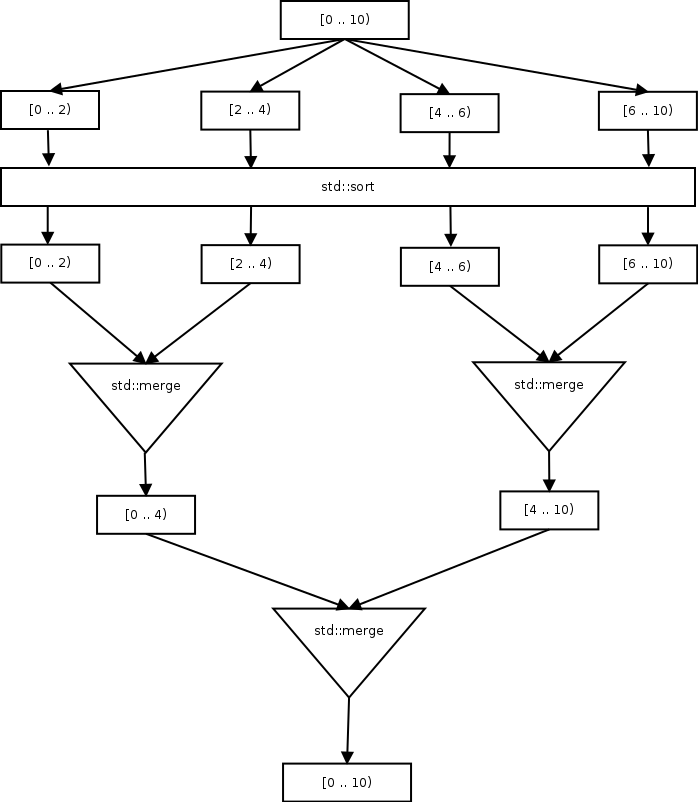We can take a concrete example to see how the algorithm works: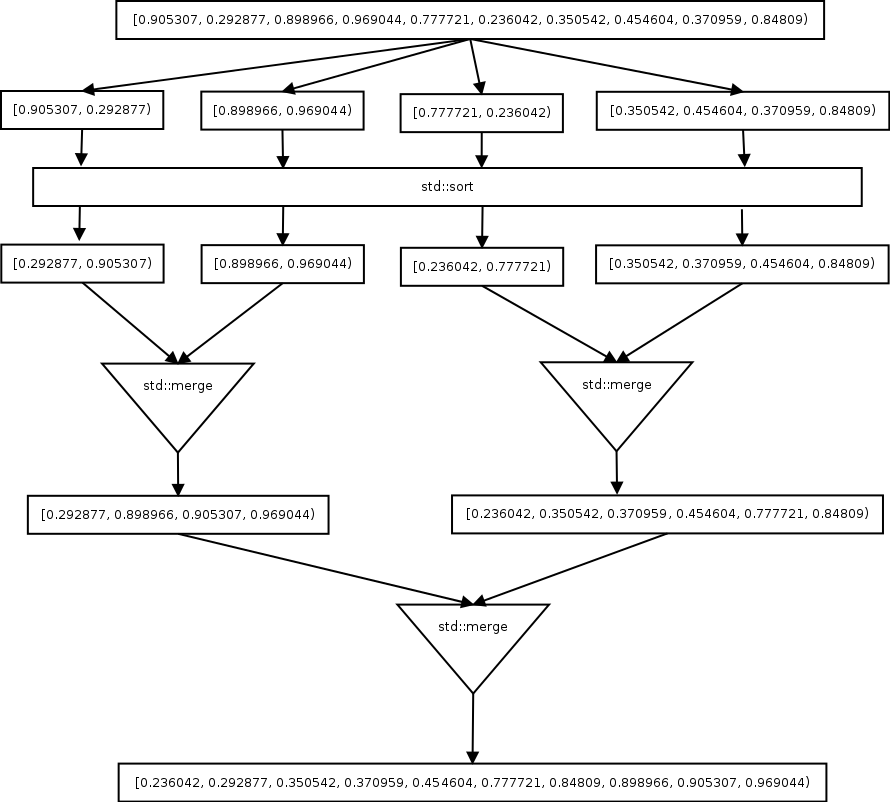In order to implement the above algorithm in C++, we start by implementing two helper functions, one for partitioning the data and one for filling an array with random numbers:

You can see the complete code for the above functions on the Github repository for this post.

Next, we list the C++ functions that implements our version of the parallel merge-sort algorithm:

run_tests will return the time, in milliseconds, in which we can sort in parallel the vector V.

The above code is 100% portable on any modern operating system that has a standard C++11 compiler.

Suppose now, that your machine has a CUDA capable GPU. What will be the easiest way to sort an array of data on the GPU ? With CUDA 5 and Thrust we can sort an array in a few lines of code:

I’ve benchmarked the above codes, CPU and GPU, on an Intel i7 Q740, 16 GB RAM, NVIDIA GeForce GT 445M with 3 GB RAM machine that runs Ubuntu 12.04. The test data was a random array of double precision numbers with 10 to 10 millions elements. The CPU code was tested in serial (single thread) and parallel (eight threads) mode.

I’ve used three C++ compilers: gcc-4.6.3 which is the default compiler on Ubuntu 12.04, gcc-4.7.2 and Clang-3.3svn with libc++. If you are interested in building from sources gcc-4.7.2 and Clang-3.3 you can consult this and this. Each test was repeated one hundred times, the data was averaged and we’ve calculated the standard deviation.

Next image presents the raw data - time versus number of elements for CPU 1/8 threads and for the GPU: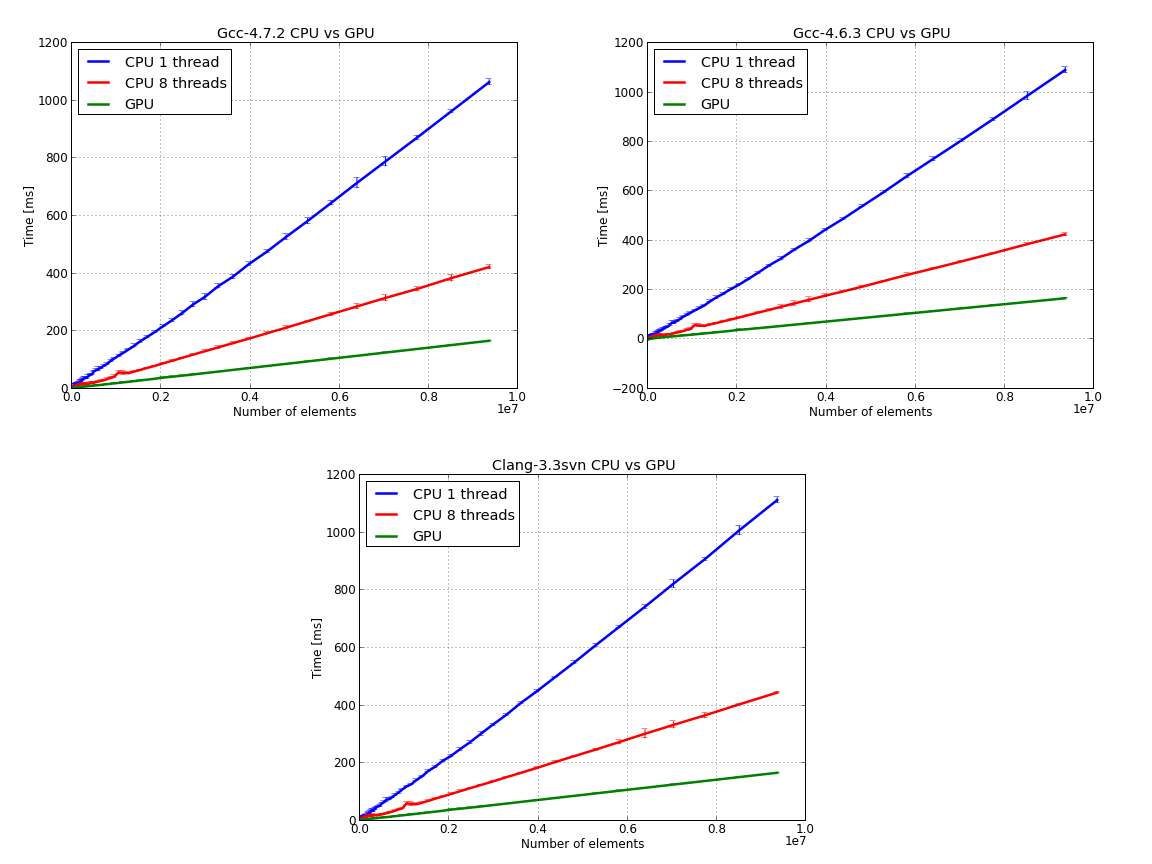Here, we’ve used the GPU time to normalize the data for the CPU:Next figure presents the data for CPU only, where we’ve used the parallel data to normalize the results: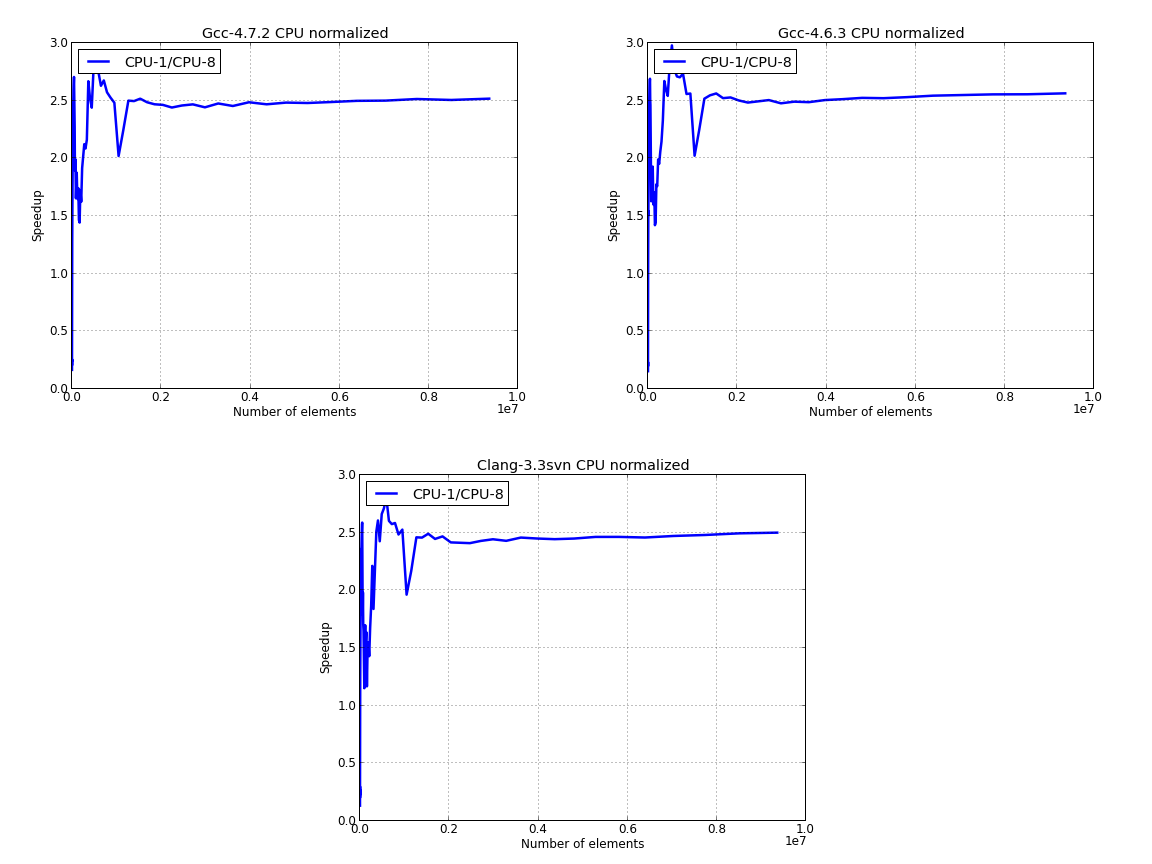The last image suggests that using the building blocks of the STL we can obtain about 2.5 speedup for sorting data in parallel versus the serial version.

In the next table, we present the size in bytes of the executable generated by each compiler:

 Compiler Gcc-4.7.2 Gcc-4.6.3 Clang-3.3svn Nvcc Executable size [bytes] 36926 48860 25575 826378

I’ve received a few messages about not taking into consideration the cost of moving data from CPU to GPU and the other way around. Your complaints were heard. In the next set of figures the GPU time contains the cost of moving the data back and forth. Also, I’ve replaced the data for the single thread test with a single call to std::sort. The original and the modified code is on Github. Without further ado let’s see the figures.

The raw data - time versus number of elements for CPU 1/8 threads and for the GPU: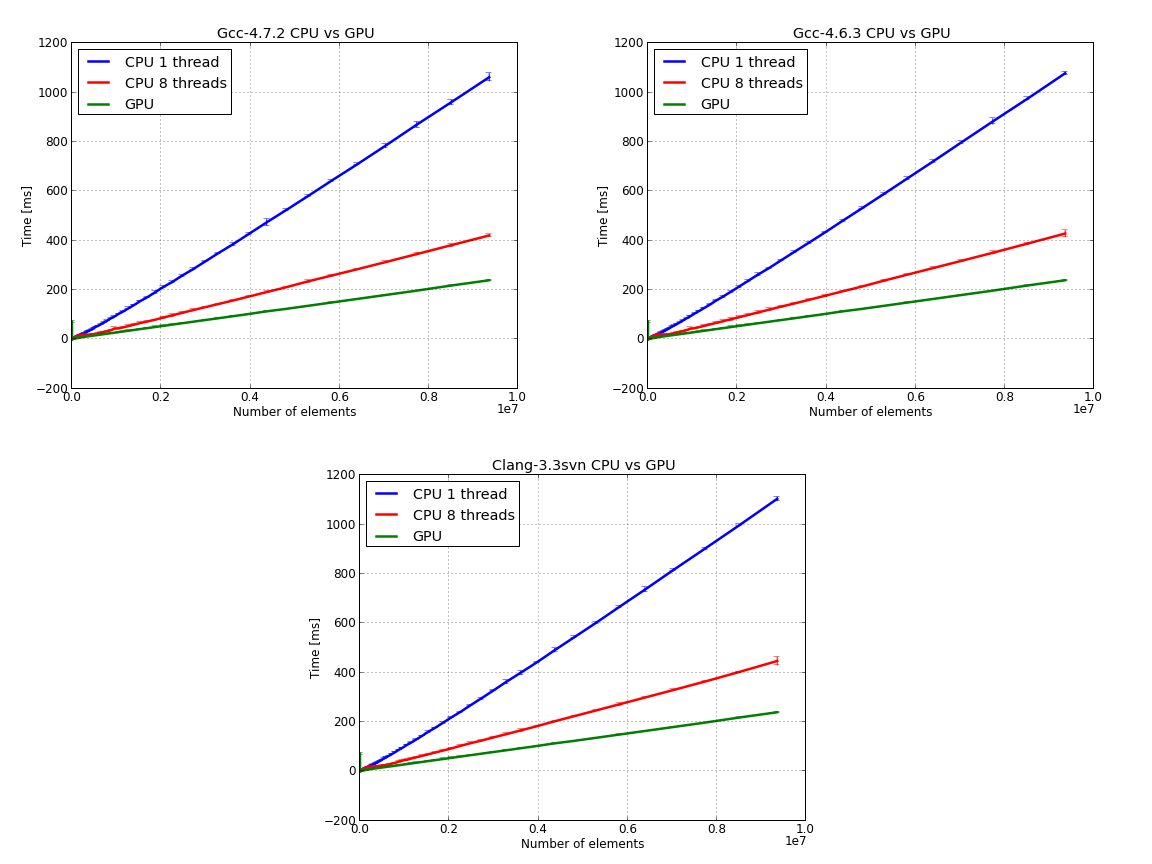Here, we’ve used the GPU time to normalize the data for the CPU: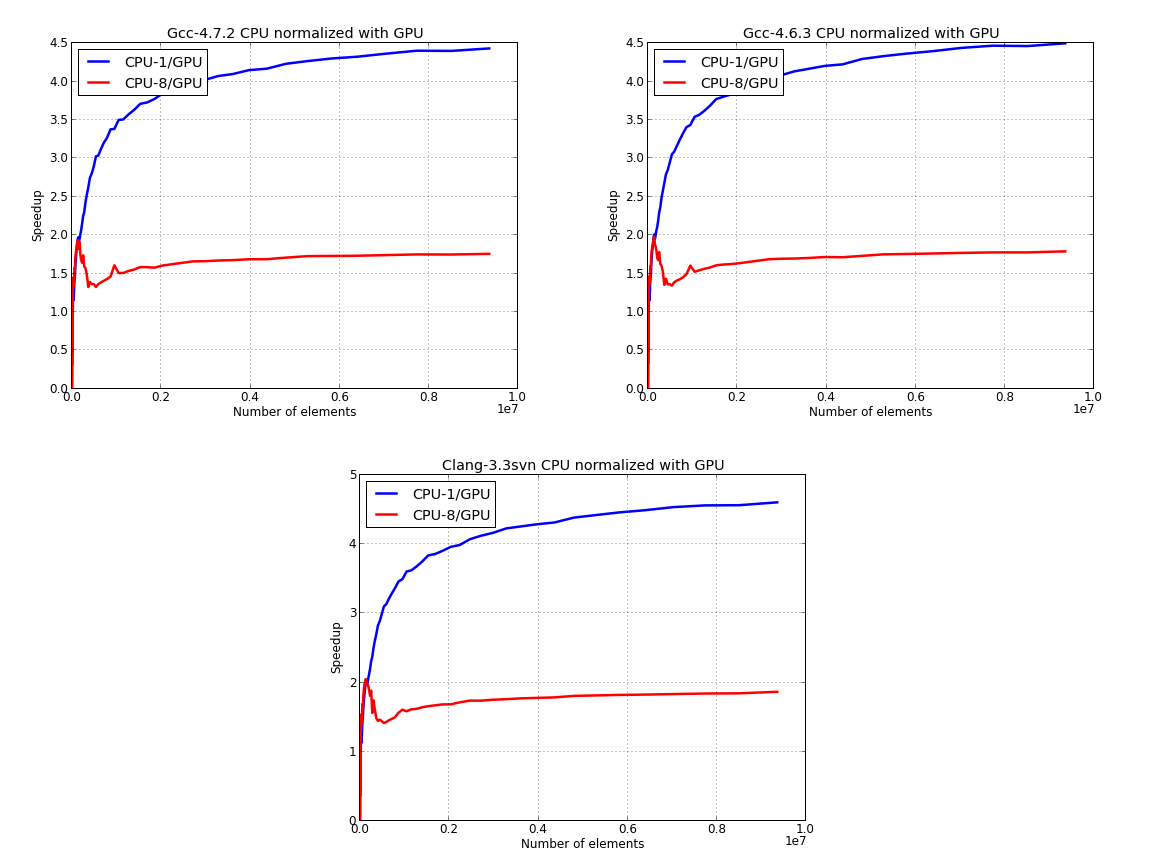Last image presents the data for CPU only, where we’ve used the parallel data to normalize the results: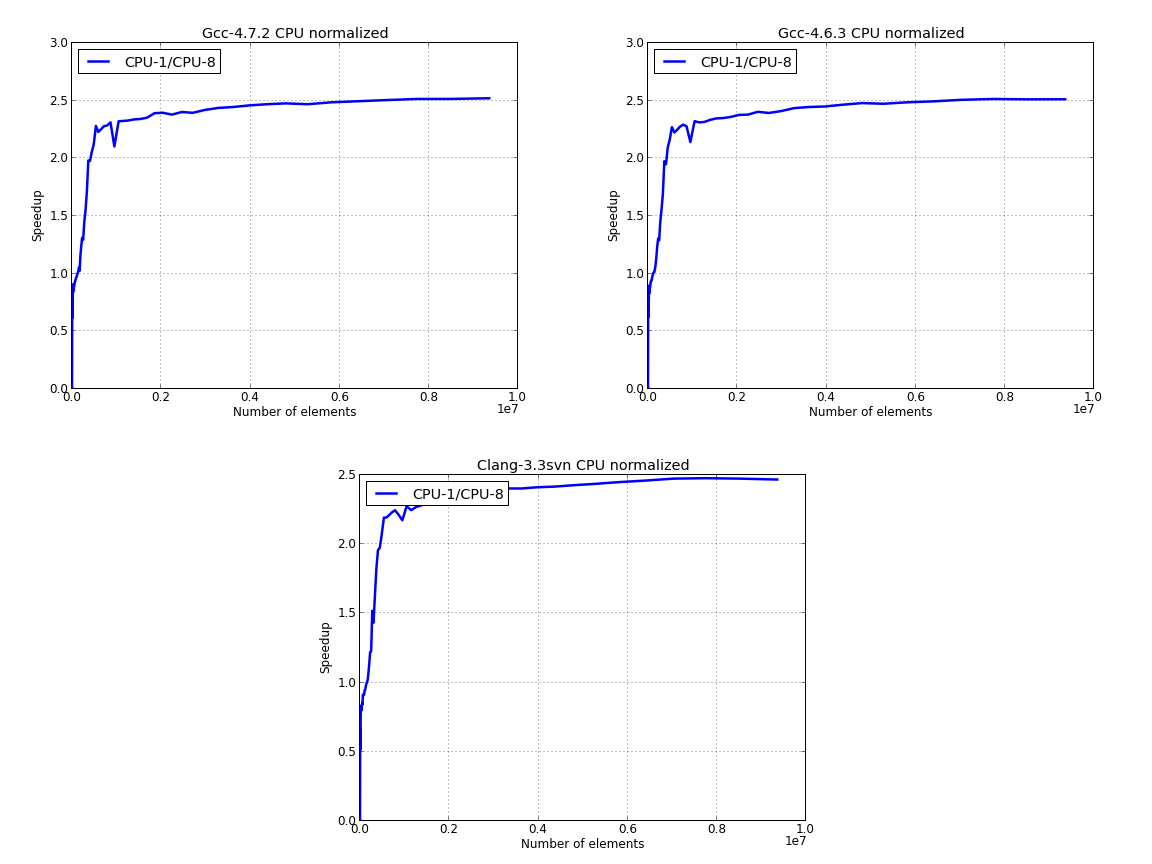If you are interested in learning more about the C++11 Standard Library, I would recommend reading The C++ Standard Library: A Tutorial and Reference (2nd Edition) by N. M. Josuttis:or Professional C++ by M. Gregoire, N. A. Solter, S. J. Kleper 2nd edition: